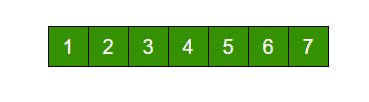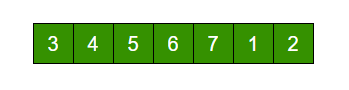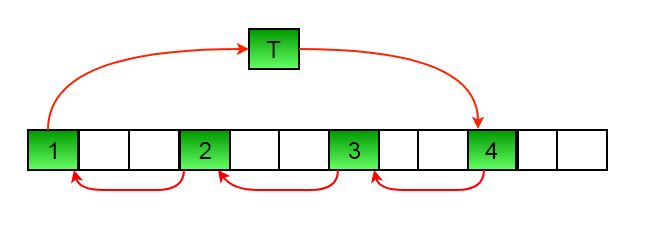Related Articles
Python Program for array rotation
• Difficulty Level : Medium
• Last Updated : 24 Mar, 2021

Write a function rotate(ar[], d, n) that rotates arr[] of size n by d elements.Rotation of the above array by 2 will make arrayMETHOD 1 (Using temp array):

```Input arr[] = [1, 2, 3, 4, 5, 6, 7], d = 2, n =7
1) Store d elements in a temp array
temp[] = [1, 2]
2) Shift rest of the arr[]
arr[] = [3, 4, 5, 6, 7, 6, 7]
3) Store back the d elements
arr[] = [3, 4, 5, 6, 7, 1, 2]```

## Python3

 `# function to rotate array by d elements using temp array``def` `rotateArray(arr, n, d):``    ``temp ``=` `[]``    ``i ``=` `0``    ``while` `(i < d):``        ``temp.append(arr[i])``        ``i ``=` `i ``+` `1``    ``i ``=` `0``    ``while` `(d < n):``        ``arr[i] ``=` `arr[d]``        ``i ``=` `i ``+` `1``        ``d ``=` `d ``+` `1``    ``arr[:] ``=` `arr[: i] ``+` `temp``    ``return` `arr`  `# Driver function to test above function``arr ``=` `[``1``, ``2``, ``3``, ``4``, ``5``, ``6``, ``7``]``print``(``"Array after left rotation is: "``, end``=``' '``)``print``(rotateArray(arr, ``len``(arr), ``2``))` `# this code is contributed by Anabhra Tyagi`
Output
`Array after left rotation is:  [3, 4, 5, 6, 7, 1, 2]`

Time complexity: O(n)
Auxiliary Space: O(d)

METHOD 2 (Rotate one by one) :

```leftRotate(arr[], d, n)
start
For i = 0 to i < d
Left rotate all elements of arr[] by one
end```

To rotate by one, store arr in a temporary variable temp, move arr to arr, arr to arr …and finally temp to arr[n-1]
Let us take the same example arr[] = [1, 2, 3, 4, 5, 6, 7], d = 2
Rotate arr[] by one 2 times
We get [2, 3, 4, 5, 6, 7, 1] after first rotation and [ 3, 4, 5, 6, 7, 1, 2] after second rotation.

## Python3

 `#Function to left rotate arr[] of size n by d*/``def` `leftRotate(arr, d, n):``    ``for` `i ``in` `range``(d):``        ``leftRotatebyOne(arr, n)` `#Function to left Rotate arr[] of size n by 1*/``def` `leftRotatebyOne(arr, n):``    ``temp ``=` `arr[``0``]``    ``for` `i ``in` `range``(n``-``1``):``        ``arr[i] ``=` `arr[i``+``1``]``    ``arr[n``-``1``] ``=` `temp``        `  `# utility function to print an array */``def` `printArray(arr,size):``    ``for` `i ``in` `range``(size):``        ``print` `(``"%d"``%` `arr[i],end``=``" "``)` ` ` `# Driver program to test above functions */``arr ``=` `[``1``, ``2``, ``3``, ``4``, ``5``, ``6``, ``7``]``leftRotate(arr, ``2``, ``7``)``printArray(arr, ``7``)` `# This code is contributed by Shreyanshi Arun`
Output
`3 4 5 6 7 1 2 `

Time complexity : O(n * d)
Auxiliary Space : O(1)

METHOD 3 (A Juggling Algorithm)
This is an extension of method 2. Instead of moving one by one, divide the array in different sets
where number of sets is equal to GCD of n and d and move the elements within sets.
If GCD is 1 as is for the above example array (n = 7 and d =2), then elements will be moved within one set only, we just start with temp = arr and keep moving arr[I+d] to arr[I] and finally store temp at the right place.
Here is an example for n =12 and d = 3. GCD is 3 and

```Let arr[] be {1, 2, 3, 4, 5, 6, 7, 8, 9, 10, 11, 12}

a)    Elements are first moved in first set – (See below diagram for this movement``````          arr[] after this step --> {4 2 3 7 5 6 10 8 9 1 11 12}

b)    Then in second set.
arr[] after this step --> {4 5 3 7 8 6 10 11 9 1 2 12}

c)    Finally in third set.
arr[] after this step --> {4 5 6 7 8 9 10 11 12 1 2 3}```

## Python3

 `#Function to left rotate arr[] of size n by d``def` `leftRotate(arr, d, n):``    ``for` `i ``in` `range``(gcd(d,n)):``        ` `        ``# move i-th values of blocks``        ``temp ``=` `arr[i]``        ``j ``=` `i``        ``while` `1``:``            ``k ``=` `j ``+` `d``            ``if` `k >``=` `n:``                ``k ``=` `k ``-` `n``            ``if` `k ``=``=` `i:``                ``break``            ``arr[j] ``=` `arr[k]``            ``j ``=` `k``        ``arr[j] ``=` `temp` `#UTILITY FUNCTIONS``#function to print an array``def` `printArray(arr, size):``    ``for` `i ``in` `range``(size):``        ``print` `(``"%d"` `%` `arr[i], end``=``" "``)`` ` `#Function to get gcd of a and b``def` `gcd(a, b):``    ``if` `b ``=``=` `0``:``        ``return` `a;``    ``else``:``        ``return` `gcd(b, a``%``b)`` ` `# Driver program to test above functions``arr ``=` `[``1``, ``2``, ``3``, ``4``, ``5``, ``6``, ``7``]``leftRotate(arr, ``2``, ``7``)``printArray(arr, ``7``)` `# This code is contributed by Shreyanshi Arun`
Output
`3 4 5 6 7 1 2 `

Time complexity : O(n)
Auxiliary Space : O(1)

Another Approach : Using List slicing

## Python3

 `# Python program using the List``# slicing approch to rotate the array``def` `rotateList(arr,d,n):``  ``arr[:]``=``arr[d:n]``+``arr[``0``:d]``  ``return` `arr``# Driver function to test above function``arr ``=` `[``1``, ``2``, ``3``, ``4``, ``5``, ``6``]``print``(arr)``print``(``"Rotated list is"``)``print``(rotateList(arr,``2``,``len``(arr))) ` `# this code is contributed by virusbuddah`
Output
```[1, 2, 3, 4, 5, 6]
Rotated list is
[3, 4, 5, 6, 1, 2]```

If array needs to be rotated by more than its length then mod should be done.

For example: rotate arr[] of size n by d where d is greater than n. In this case d%n should be calculated and rotate by the result after mod.
Please refer complete article on Program for array rotation for more details!

My Personal Notes arrow_drop_up